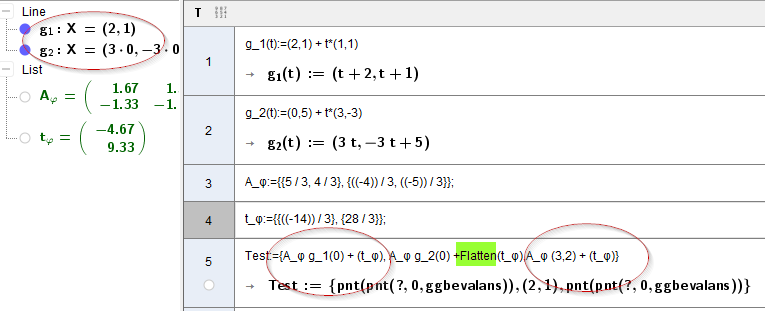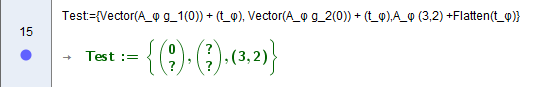# Classic CAS Line and Matrix+Vector

hawe shared this problem 2 years ago
Not a Problem

Line def in CAS has nonsense entry in AlgebraView

always problems Matrix*Vector + Vector1

Fractions In Vector like g(t):= (1,2)+t (1,1/2) will fail, no graph in grafik window1

Matrix * Point gives Point so you're trying to do Point + Matrix which doesn't work

```A_φ (3,2) + (t_φ)
```1

Hm,

but point + list works?

how to define g_1(t) as vector

( a vector def in AlgView a1=(2,1), r1=(1,1) ===> g_1(t):=a1 + t r1 will crash graph of line in grafics)

or

Test:={A_φ Vector(g_1(0)) + (t_φ),A_φ Vector(g_2(0)) + (t_φ),A_φ (3,2) +Flatten(t_φ)}

same as